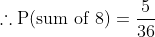# UGC NET COMMERCE SOLVED PAPERS 2017-19 - UGC NET COMMERCE June 2019

>>>>>>>>UGC NET COMMERCE June 2019

• A

(a), (b), (d) and (e)• B

(a), (c), (d) and (e)• C

(b), (c), (d) and (e)• D

(a), (b), (c) and (d)• Option : B
• Explanation : The outcomes of favourable to the event ‘sum of two numbers is 8’ are (6, 2), (5, 3), (4, 4), (3, 5) and (2, 6). There are 5 in number:• A

inelastic production• B

negative marginal production• C

declining total production• D

declining average production• Option : B
• Explanation : The law of diminishing marginal returns states that as more of a variable resource is combined with a given amount of another resource, marginal product eventually declines. The law of diminishing marginal returns is the most important feature of production in the short run.

• A

Rs.4,80,000• B

Rs.5,00,000• C

Rs.4,20,000• D

Rs.4,50,000• Option : A
• Explanation : Step-1 : Higher of Municipal value and Fair Rent in this case it will be higher of 450,000 and 5,00,000, So it will be 5,00,000 (let us call it as A).
Step 2 : Lower of A and Standard Rent so it will be lower of 5,00,000 and 4,80,000, So will be 4,80,000. (let us call it B)
Step 3 : Higher of ‘B’ and Actual Rent, So it will be Higher of 4,80,000 and 4,20,000. So the GAV will be 4,80,000.